View Course Path

# Internal Scan Chain – Structured techniques in DFT (VLSI)

Contents

## DFT in Sequential Circuits

Earlier in this DFT course, we learnt a few test generation techniques in combinational circuits. But their major drawback was, they weren’t suitable for sequential circuits. From this article onwards, we will discuss the industry-grade techniques employed in Design for Testability.

### ATPG Problem in Sequential Circuits

Consider a sequential circuit, as shown below. It consists of two flip-flops and several gates connected to each other. This circuit has a single input and output, just like an inverter. But it is not a simple circuit as an inverter. This is a mealy state machine, whose next states as well as output depends upon the input in. We need to be careful while testing this circuit.

Let’s consider a `s-a-0` fault (stuck-at-zero fault), as shown in the diagram. For a node to be tested, it should be controllable as well as observable. But none of these nodes satisfies this condition. The nodes closer to the input can be controllable, but not observable easily since they are passed through a layer of FFs and combinational circuit. While the nodes after the FF Q pin can be observable since they are closer to output, but aren’t controllable directly.

Of course, some of these nodes can be controllable and observable if we find a technique to initialize the flip-flops to the desired value and propagate the fault by the path sensitization approach. But this is a complicated approach.

Hence combinational ATPG techniques like D-algorithm or PODEM can’t be applied in sequential circuits. It is a significant problem in testing that internal flip-flops can’t be easily controlled or observed.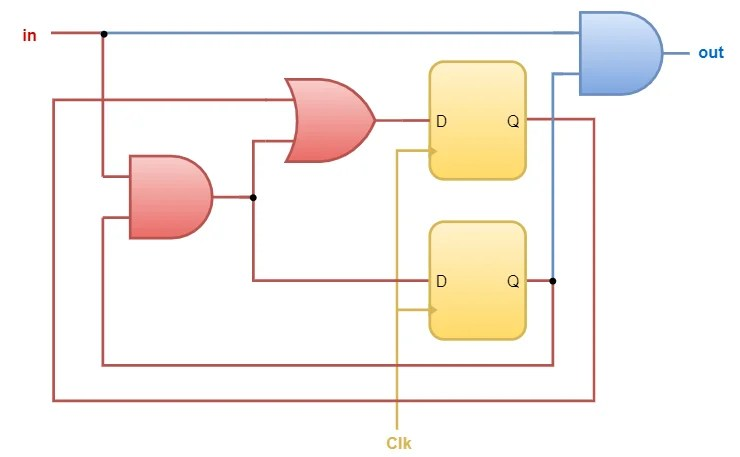### Sequential Circuit Partition

So how should we address this problem? Let’s partition this circuit. The above circuit can be divided into three different parts. The logic gates in red represent the next-state combinational circuit, and those in blue represent the output combinational circuit. This is a mealy state machine; hence we can observe the primary input is also connected to the output combinational circuit. The Flip-flops or storage elements are represented in yellow. Let’s drag the FFs outside from the sequential circuit, as shown in the figure below.The red and blue parts can be combined to represent a part of a bigger combinational circuit. Hence, every sequential circuit can be represented in this way. The inputs to the combinational circuit from the FFs are called as pseudo-primary inputs, while the outputs of the combinational circuit to FFs are called pseudo-primary outputs.

### Controllability and Observability Issues

The flip-flops are the main culprits that make sequential circuit testing difficult. The idea is to separate the flip-flops from the rest of the circuit so that the combinational part can be tested easily using ATPG.

Now, if we can control and observe these pseudo inputs and outputs to the combinational circuit, we can easily test this circuit with previously discussed ATPG methods. Hence, we don’t need to worry about flip-flops. This is the essence of Internal Scan Design.

But how to control or observe these pseudo-primary inputs or pseudo-primary outputs? For this, we need to replace the standard flip-flops with a special type of flip-flop through which we can initialize and control from outside the chip.

#### Scan Flip-Flop

Here’s a typical implementation of the Scan Flip-Flop (SFF) using a normal Flip-Flop.Muxed-D Scan Flip Flop, as the name suggests, this is a conventional flip-flop with a 2:1 MUX before it. This additional feature allows the flip-flop to be initialized with any value by setting the Scan Enable Pin. Scan Flip-Flop has four main pins:

• Scan Chain: Scan In (SI), Scan Out (SO)
• Logic: Data In (DI), Data Out (DO)

DO and SO pins are shared, as shown in the diagram. The Scan Enable pin is the select line to the multiplexer, which selects between the Data In and the Scan In input. The DFF captures the value, which is decided by the Scan Enable pin. Scan Flip-Flop has two functions:

• Shift data from SI pin to SO pin (when SE = 1)
• Capture data from the combinational circuit (when SE = 0)

#### Scan Chain

Let’s incorporate this new flip-flop into our design.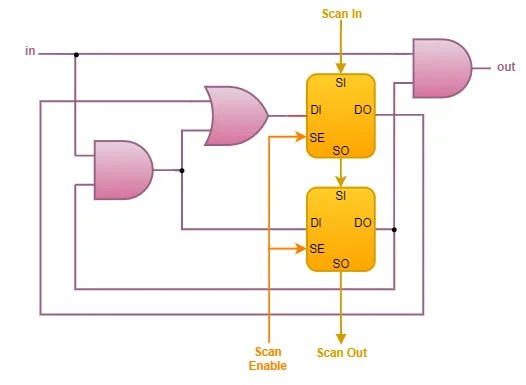In the modified design, the scan flip-flops are stitched together one after another in a Scan Chain. In the previous circuit, the outputs of the combinational circuit were directly connected to the flip-flop inputs. But, in the newer design, we have disconnected the line using a multiplexer. Now, we have the choice to either connect the combinational circuit output to the flip flop inputs (Normal Mode) or to connect it to Scan In pin (Test Mode). Hence the circuit now has two operation modes: Normal mode and Test mode.

#### DFT Insertion

The idea of the Internal Scan is to connect internal Flip-Flops and latches so that we can observe them in test mode. Scan remains one of the most popular structured techniques for digital circuits. This above process is known as Scan chain Insertion. In the VLSI industry, it is also known as DFT Insertion or DFT synthesis.

The steps involved in DFT synthesis are:

• Replace FF/latch
• Stitch FF/latch into a chain

## Modes of operation in Scan Chain

As previously discussed, Scan Chain operates in two modes.

### Normal Mode

• In normal mode, Scan Flip-Flops are configured to perform capture operation.
• They capture the response from the logic and then apply the response to the logic in the next clock cycle.
• The Normal Mode is activated when Scan Enable is logic-o.
• The flip-flops capture the data from pseudo-primary outputs of the combinational logic.
• This mode is exactly similar to the original mode, and the Scan flip-flops can be simply ignored in this case in lieu of normal flip-flops.

### Test Mode

• In test mode, the scan flip-flops are first configured to perform shift operation so we can shift-in our test pattern.
• And then the scan flip-flops are configured to capture the response from the logic.
• Finally, we configure the flip-flops to perform the shift-out operation so that we can observe the values in the Scan flip-flops.
• The following steps are involved in test mode:
Step 1: Shift In

The Test Mode is activated when Scan Enable is logic-1. We now have the ability to initialize the FFs. Notice in the figure, the SI pin of the first FF is connected to the SO pin of the second FF. Hence, the two FFs behaves like a shift register. Note, that SO pin is simply the Q pin of the DFF because SO and DO are shorted together. This is a 2-bit shift register. For n no. of FFs, it would behave like an n-bit shift register. In this way, we could shift data serially to each FFs using the Scan In pin of the first FF. This solves the controllability issue of the pseudo-primary inputs.

Step 2: Capture

When all the FFs are initialized to their desired value (corresponding to the test vector for pseudo-primary inputs), the circuit is then operated in Normal Mode for one clock cycle. The Scan Enable Pin is set low. In this phase, the combinational logic undergoes a test. The outputs are captured in respective FFs in the next clock cycle. Now, the FF values are replaced with the result of the test vectors from respective pseudo primary outputs. Note that in the combinational circuit, the total inputs consist of pseudo-primary inputs as well as the primary input. So, in addition to the shifting inputs for pseudo-primary inputs, we also need to apply the test vector to the primary inputs at the same time.

Step 3: Shift Out

When the testing is done, we need to shift out the results from the FFs so that we can examine them. The Scan Enable is set to logic-1 again. This is a similar process to Step 1, but in this case, instead of shifting in, the FF data is shifted out. This solves the observability issue of the pseudo-primary outputs.

## Test Generation for Scan Chains

Let’s demonstrate the entire process with a few examples.

### Example 1

Take the previous circuit. Say we need to test the `s-a-1` fault present in the circuit. This is a fanout branch; hence it is a checkpoint which can’t be collapsed. First, let’s try to test this circuit in normal mode using the path sensitization method.Fault excitation requires the output of G1 to be logic-1; this can only be made by applying both the inputs of AND gate G1 to logic-1. Applying logic-1 to 1st input is not an issue since it is directly controlled by input. But setting the 2nd input of the G1 gate is not easy since it is connected to the DO pin of D2 SFF.

Let’s not worry, just backpropagate.

For D2 DO to be set logic-1, we need to set D2 DI to logic-1 in the previous clock cycle. This pin is connected to G1 out. We just arrived at the same point. By back-propagating further, we would simply loop around. So, you can see that even exciting this fault is a nightmare.

There are two more steps, forward and backward propagation too. Hence, there is no choice; we need to use the Internal Scan technique only.

Let’s just focus on the combinational circuit of interest and apply the path sensitization approach to generate the test vector.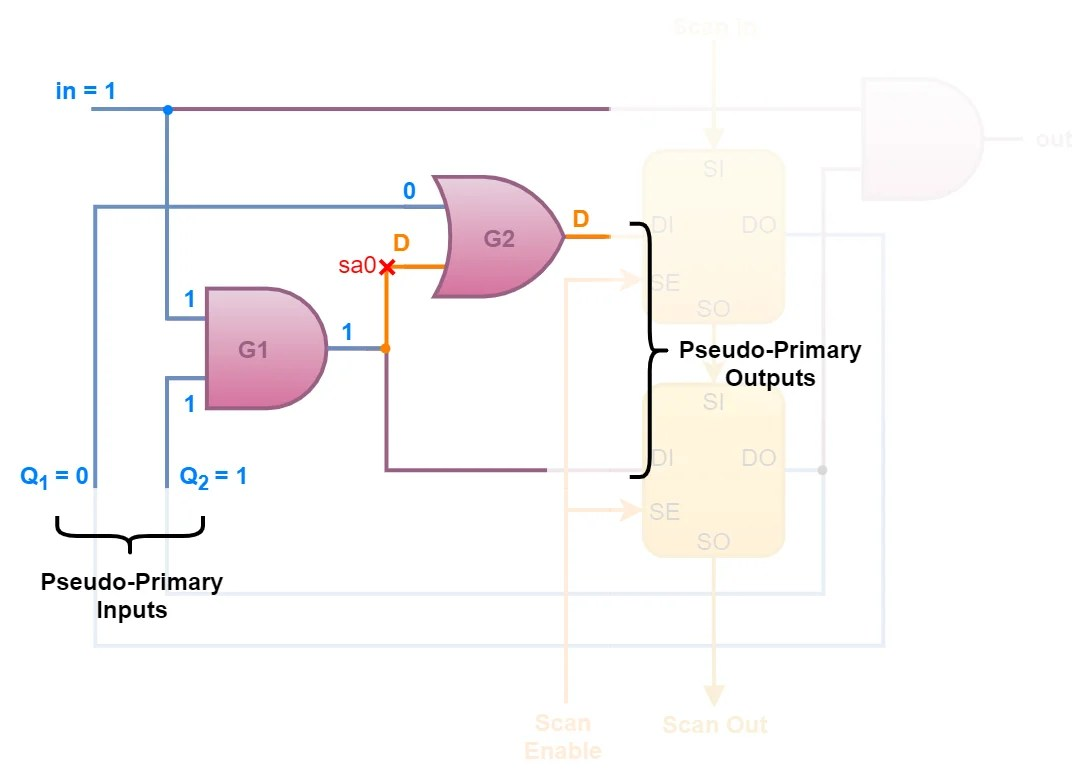We can observe that, in and G1, both inputs need to be initialized to logic-1 for fault excitation. Hence Q2 and in must be set logic-1. For forward propagation in the next level OR gate G2, Q1 should be applied logic-0. Back-propagation is not required since all primary as well as pseudo-inputs are known. The test vector is `{in, Q1, Q2} = {1, 0, 1}`. Let’s move into the Internal Scan architecture required for testing.

Step 1: Shift InApply SE as logic-1 to disconnect the FFs from the state machine and enter into the test mode. We serially insert the test vector `{Q2, Q1} = {1, 0}`. The test vector requires two clock cycles to initialize the two FFs. Also, don’t forget to initialize in to logic-1. The fault is successfully excited.

Step 3: Shift Out

To observe the output result, we need to re-enter the shift mode. The SE pin is set logic-1. The first shift gets us the value of Q2, which is utterly useless for this example. The next shift yields the discrepancy D. By examining the value of D, we can quickly test for the `s-a-0` fault.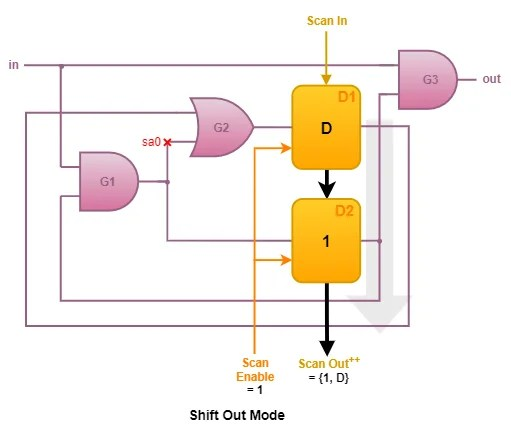If D is logic-0, then the circuit is `s-a-0` faulty; otherwise, the circuit is free from that fault. Following is the waveform of the scan process.As we can see from the example, the Scan turns a sequential circuit into a combinational circuit problem because the flip-flops are fully scannable. Due to this, the pseudo-primary inputs and outputs are entirely controllable as well as observable. Hence ATPG sees only the combinational circuit model.

### Example 2

Let’s do another example; consider the sequential circuit below. We need to test the `sa1` and `sa0` faults in the circuit.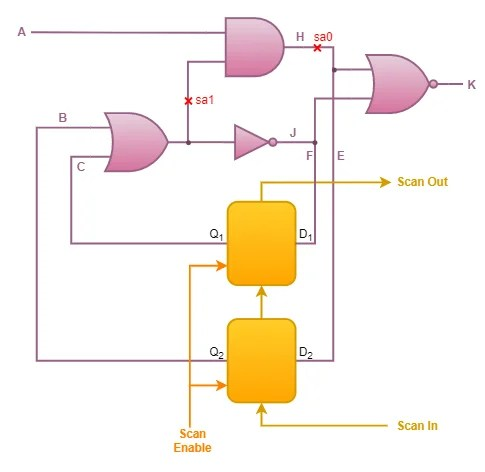We can extract the combinational part and apply ATPG techniques to generate test patterns. Considering the single stuck-at fault model, we need to test the two faults separately using two different test patterns. The respective test pattern for `sa0` and `sa1` faulty circuit is shown below.We obtain the combinational test pattern as two test vectors which can be represented as `{A, B, C} = {(1, 1, x), (1, 0, 0)}`. The x is don’t care. But this combinational pattern can’t be applied on a sequential circuit. We need to convert it into a scan pattern. The scan pattern is summarized in a tabular form below.

 Test Vector Step 1 (Shift In) Step 2 (Capture) Step 3 (Shift Out + Shift In) Step 4 (Capture) SE = 1 SE = 0 SE = 1 SE = 0 {A, B, C} = {1, 1, x} SI = {x, 1} A = 1 D2 = D SO = {0, D} {A, B, C} = {1, 0, 0} SI = {0, 0} A = 1 D2 = D K = D’
Steps

Step 1: For `{A, B, C} = {1, 1, x}`, since B and C are pseudo-primary inputs, we need to shift in `{1, x}` for BC to get initialized. Note the entities in curly braces ( {} ) are shifted from left to right. Since A is the primary input, we can feed logic-1 directly to A. Don’t forget while doing this, SE needs to be enabled. The fault has been excited and propagated at this step.

Step 2: To capture the test results, SE is disabled. D is stored in Q2 of the 2nd SFF.

Step 3: Now, to unload the results, we need to shift only two times; since the result is stored in the very first SFF of the chain. The SE is enabled. D is shifted out. At the same time, we also cleverly shift in `{0, 0}` for the 2nd test vector to load `BC = {0, 0}`. This saves testing time. During unloading the results, we are simultaneously loading the values for the next test.

Step 4: To capture the test results, SE is again disabled. D is stored in Q2 of the 2nd SFF.

But wait, do we need to shift out again? Notice that D’ is already available at the primary output K. Hence in this circuit, we don’t need to shift out the results.

In the previous case, we could also extract D’ from primary output K. But previous shift operation was compulsory, as we again need to shift in the next test vector.

In the case of the last test vector, we could save time by skipping the shift-out operation. Following is the waveform of the above operations.## Pros and Cons of Internal Scan

• Systematic DFT, not ad hoc, there are many automatic CAD tools available to insert Scan Chain IP into the design automatically.
• It makes ATPG easier so that run time is faster and fault coverage higher.
• The scan chain facilitates silicon debugging or diagnosis for prototype and pre-production chips.

But there is no free lunch. Structured techniques like Internal Scan suffer from various disadvantages.

• Area overhead due to extra elements in Scan flip-flops and routing for Scan Chains. In industry, typically, 5-10% overhead is acceptable.
• Performance overhead because Scan flip-flop has a longer setup time and hold time. This decreases the max rated frequency.
• Pin overhead in IC due to pins like Scan In, Scan Out, Scan Enable, Scan Clocks, etc.
• Power overhead in normal operation due to static power dissipation in the additional testing circuitry.
• It requires extra design efforts in VLSI flow for Scan Chain insertion and its verification.

## Summary

In this section, we came across how Internal Scan facilitates the application of conventional ATPG test patterns to the sequential circuit by simplifying them into a combinational circuit, preserving the original circuit functionality with minimal circuit overhead. This is done by replacing the traditional flip-flops with Scan flip-flops and connecting them in a Scan Chain. We studied Scan flip-flop implementation using the muxed-D technique. But there are more alternative and efficient ways to implement the Scan flip-flop. In the next section, we will go through various other implementation of the Scan flip-flops based on their applications.

Related courses for this will be up soon!

This site uses Akismet to reduce spam. Learn how your comment data is processed.

Top